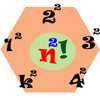#### You may also like### Growing

Which is larger: (a) 1.000001^{1000000} or 2? (b) 100^{300} or 300! (i.e.factorial 300)### Seriesly

Prove that k.k! = (k+1)! - k! and sum the series 1.1! + 2.2! + 3.3! +...+n.n!### Factorial Fun

How many divisors does factorial n (n!) have?

# Seriesly

##### Age 16 to 18 Short Challenge Level:

Prove that
$$k \times k! = (k+1)! - k!$$ and sum the series
$$1 \times 1! + 2 \times 2! + 3 \times 3! +...+n \times n!$$

Did you know ... ?
A telecoping series is a series that can be written as the difference of two expressions in such a way that almost all the terms cancel with the following or preceding term leaving a few terms which can be combined to give the sum of the series. See the Wikipedia article Telescoping Series.